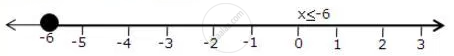# Represent the Solution of the Given Inequalities on the Real Number Line X + 3 <= 2x + 9 - Mathematics

Represent the solution of the given inequalities on the real number line

x + 3 <= 2x + 9

#### Solution

x + 3 <= 2x + 9

-6 <= x

The solution on number line isConcept: Linear Inequations in One Variable
Is there an error in this question or solution?

#### APPEARS IN

Selina Concise Maths Class 10 ICSE
Chapter 4 Linear Inequations (In one variable)
Exercise 4 (B) | Q 4.3 | Page 49